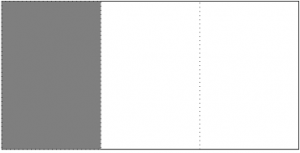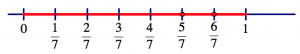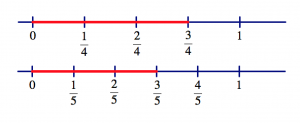Skip to main content
$$\newcommand{\id}{\mathrm{id}}$$ $$\newcommand{\Span}{\mathrm{span}}$$ $$\newcommand{\kernel}{\mathrm{null}\,}$$ $$\newcommand{\range}{\mathrm{range}\,}$$ $$\newcommand{\RealPart}{\mathrm{Re}}$$ $$\newcommand{\ImaginaryPart}{\mathrm{Im}}$$ $$\newcommand{\Argument}{\mathrm{Arg}}$$ $$\newcommand{\norm}{\| #1 \|}$$ $$\newcommand{\inner}{\langle #1, #2 \rangle}$$ $$\newcommand{\Span}{\mathrm{span}}$$

# 4.5: What is a Fraction? Revisited

•• Contributed by Michelle Manes
• Professor (Mathematics) at University of Hawaii

$$\newcommand{\vecs}{\overset { \rightharpoonup} {\mathbf{#1}} }$$

$$\newcommand{\vecd}{\overset{-\!-\!\rightharpoonup}{\vphantom{a}\smash {#1}}}$$

So far, we have been thinking about a fraction as the answer to a division problem. For example, $$\frac{2}{3}$$ is the result of sharing two pies among three children.Of course, pies do not have to be round. We can have square pies, or triangular pies or squiggly pies or any shape you please.This “Pies Per Child Model” has served us perfectly well in thinking about the meaning of fractions, equivalent fractions, and even adding and subtracting fractions.

However, there is no way to use this model to make sense of multiplying fractions! What would this mean?So what are fractions, if we are asked to multiply them? We are forced to switch models and think about fractions in a new way.

This switch is fundamentally perturbing. Think about students learning this for the first time. We keep switching concepts and models, and speak of fractions in each case as though all is naturally linked and obvious. None of this is obvious, it is all absolutely confusing. This is just one of the reasons that fractions can be such a difficult concept to teach and to learn in elementary school!

Think / Pair / Share (What’s wrong here?)

For each of the following visual representations of fractions, there is a corresponding incorrect symbolic expression.

• Why is the symbolic representation incorrect?
• What might elementary students find confusing in these visual representations?$$\frac{1}{3} \qquad \qquad \frac{2}{3} > \frac{3}{4}$$$$\frac{1}{3} \neq 13$$

## Units and unitizing

In thinking about fractions, it is important to remember that there are always units attached to a fraction, even if the units are hidden. If you see the numberin a problem, you should ask yourself “half of what?” The answer to that question is your unit, the amount that equals 1.

So far, our units have been consistent: the “whole” (or unit) was a whole pie, and fractions were represented by pies cut into equal-sized pieces. But this is just a model, and we can take anything, cut it into equal-sized pieces, and talk about fractions of that whole.

One thing that can make fraction problems so difficult is that the fractions in the problem may be given in different units (they may be “parts” of different “wholes”).

Example (Everyone is right!)

Mr. Li shows this picture to his class and asks what number is shown by the shaded region.• Kendra says the shaded region represents the number 5.
• Dylan says it represents $$2 \frac{1}{2}$$.
• Kiana says it represents $$\frac{5}{8}$$.
• Nate says it is $$1 \frac{1}{4}$$.

Mr. Li exclaims, “Everyone is right!”

Think / Pair / Share

1. How can it be that everyone is right? Justify each answer by explaining what each student thought was the unit in Mr. Li’s picture.
2. Now look at this picture:• If the shaded region represents $$3 \frac{2}{3}$$, what is the unit?
• Find three other numbers that could be represented by the shaded region, and explain what the unit is for each answer.

Example (Segments)

This picturerepresents $$\frac{2}{3}$$. The whole segment (the unit) is split into three equal pieces by the tick marks, and two of those three equal pieces are shaded.

Think / Pair / Share

For each picture below, say what fraction it represents and how you know you are right.## Ordering Fractions

If we think about fractions as “portions of a segment,” then we can talk about their locations on a number line. We can start to treat fractions like numbers. In the back of our minds, we should remember that fractions are always relative to some unit. But on a number line, the unit is clear: it is the distance between 0 and 1.This measurement model makes it much easier to tackle questions about the relative size of fractions based on where they appear on the number line. We can mark off different fractions as parts of the unit segment. Just as with whole numbers, fractions that appear farther to the right are larger.3/5 and 5/8 are very close, but 5/8 is just a bit bigger.

Think / Pair / Share (Ordering fractions)

1. What quick method can you use to determine if a fraction is greater than 1?
2. What quick method can you use to determine if a fraction is greater than $$\frac{1}{2}$$?
3. Organize these fractions from smallest to largest using benchmarks: 0 to $$\frac{1}{2}$$, $$\frac{1}{2}$$ to 1, and greater than 1. Justify your choices. $$\frac{25}{23}, \quad \frac{4}{7}, \quad \frac{17}{35}, \quad \frac{2}{9}, \quad \frac{14}{15} \ldotp$$
4. Arrange each group of fractions in ascending order. Keep track of your thinking and your methods.
• $$\frac{7}{17}, \quad \frac{4}{17}, \quad \frac{12}{17} \ldotp$$
• $$\frac{3}{7}, \quad \frac{3}{4}, \quad \frac{3}{8} \ldotp$$
• $$\frac{5}{6}, \quad \frac{7}{8}, \quad \frac{3}{4} \ldotp$$
• $$\frac{8}{13}, \quad \frac{12}{17}, \quad \frac{1}{6} \ldotp$$
• $$\frac{5}{6}, \quad \frac{10}{11}, \quad \frac{2}{3} \ldotp$$

You probably came up with benchmarks and intuitive methods to think about the relative sizes of fractions. Here are some of these methods. (Did you come up with others?)

## Fraction Intuition

Greater than 1: A fraction is greater than 1 if its numerator is greater than its denominator. How can we see this? Well, the denominator represents how many pieces in one whole (one unit). The numerator represents how many pieces in your portion. So if the numerator is bigger, that means you have more than the number of pieces needed to make one whole.Greater than $$\frac{1}{2}$$: A fraction is greater than $$\frac{1}{2}$$ if the numerator is more than half the denominator. Another way to check (which might be an easier calculation): a fraction is greater than $$\frac{1}{2}$$ if twice the numerator is bigger than the denominator.

Why? Well, if we double the fraction and get something bigger than 1, then the original fraction must be bigger than $$\frac{1}{2}$$.

Same denominators: If two fractions have the same denominator, just compare the numerators. The fractions will be in the same order as the numerators. For example, $$\frac{5}{7} < \frac{6}{7}$$. Why? Well, the pieces are the same size since the denominators are the same. If you have more pieces of the same size, you have a bigger number.Same numerators: If the numerators of two fractions are the same, just compare the denominators. The fractions should be in the reverse order of the denominators. For example, $$\frac{3}{4} > \frac{3}{5}$$. The justification for this one is a little trickier: The denominator tells you how many pieces make up one whole. If there are more pieces in a whole (if the denominator is bigger), then the pieces must be smaller. And if you take the same number of pieces (same numerator), then the bigger piece wins.Numerator = denominator$$-1$$: You can easily compare two fractions whose numerators are both one less than their denominators. The fractions will be in the same order as the denominators. Think of each fraction as a pie with one piece missing. The greater the denominator, the smaller the missing piece, so the greater the amount remaining. For example, $$\frac{6}{7} < \frac{10}{11}$$, since $$\frac{6}{7} = 1 - \frac{1}{7}$$ and $$\frac{10}{11} = 1 - \frac{1}{11}$$.Numerator = denominator − constant: You can extend the test above to fractions whose numerators are both the same amount less than their denominators. The fractions will again be in the same order as the denominators, for exactly the same reason. For example, $$\frac{3}{7} < \frac{7}{11}$$, because both are four “pieces” less than one whole, and the $$\frac{1}{11}$$ pieces are smaller than the $$\frac{1}{7}$$ pieces.

Equivalent fractions: Find equivalent fractions that lets you compare numerators or denominators, and then use one of the above rules.

## Arithmetic Sequences

Consider the patterns below.

Pattern 1: $$5, \quad 8, \quad 11, \quad 14, \quad 17, \quad 20, \quad 23, \quad 26, \ldots$$

Pattern 2: $$2, \quad 9, \quad 16, \quad 23, \quad 30, \quad 37, \quad 4, \quad 51, \ldots$$

Pattern 3: $$\frac{1}{5}, \quad \frac{3}{5}, \quad 1, \quad \frac{7}{5}, \quad \frac{9}{5}, \quad \frac{11}{5}, \quad \frac{13}{5}, \quad 3, \ldots$$

Think / Pair / Share

Answer these questions about each of the patterns.

• Can you predict the next five numbers?
• Can you predict the 100th number?
• What do these sequences have in common? Describe the pattern in words.

The patterns above are called arithmetic sequences: a sequence of numbers where the difference between consecutive terms is a constant. Here are some other examples:

Pattern A: $$\begin{split} \underbrace{1, \quad} \underbrace{2, \quad} \underbrace{3, \quad} \underbrace{4, \quad} 5, & \ldots \\ +1\; \; +1\; \; +1\; \; +1 \quad & \end{split}$$

Pattern B: $$\begin{split} \underbrace{2, \quad} \underbrace{4, \quad} \underbrace{6, \quad} \underbrace{8, \quad} 10, & \ldots \\ +2\; \; +2\; \; +2\; \; +2 \qquad & \end{split}$$

Pattern C: $$\begin{split} \underbrace{\frac{1}{3}, \quad} \underbrace{1, \quad} \underbrace{\frac{5}{3}, \quad} \underbrace{\frac{7}{3}, \quad} 3, & \ldots \\ + \frac{2}{3}\; \; + \frac{2}{3}\; + \frac{2}{3}\; \; + \frac{2}{3}\; \quad & \end{split}$$

Think / Pair / Share

If you have not done so already, find the common difference between terms for Patterns 1, 2, and 3. Are they really arithmetic sequences?

Then make up your own arithmetic sequence using whole numbers. Exchange sequences with a partner, and check if your partner’s sequence is really an arithmetic sequence.

Here are several more number patterns:

Pattern 4: $$1, \quad 2, \quad 4, \quad 8, \quad 16, \quad 32, \quad 64, \quad 128, \ldots$$

Pattern 5: $$1, \quad 3, \quad 6, \quad 10, \quad 15, \quad 21, \quad 28, \quad 36, \ldots$$

Pattern 6: $$\frac{2}{5}, \quad \frac{7}{10}, \quad 1, \quad \frac{13}{10}, \quad \frac{8}{5}, \quad \frac{19}{10}, \quad \frac{11}{5}, \quad \frac{5}{2}, \ldots$$

Pattern 7: $$\frac{3}{5}, \quad \frac{6}{5}, \quad \frac{12}{5}, \quad \frac{24}{5}, \quad \frac{48}{5}, \quad \frac{96}{5}, \ldots$$

Think / Pair / Share

For each of the sequences above, decide if it is an arithmetic sequence or not. Justify your answers.

Problem 2

$$\frac{1}{4}, \quad \_\_\_\_, \quad \_\_\_\_, \quad \frac{1}{3}$$

1. Find two fractions between $$\frac{1}{4}$$ and $$\frac{1}{3}$$.
2. Are the resulting four fractions in an arithmetic sequence? Justify your answer.

Problem 3

Find two fractions between $$\frac{1}{6}$$ and $$\frac{1}{5}$$ so the resulting four numbers are in an arithmetic sequence.

$$\frac{1}{6}, \quad \_\_\_, \quad \_\_\_\_, \quad \frac{1}{5}$$

Problem 4

Find three fractions between $$\frac{2}{5}$$ and $$\frac{5}{7}$$ so the resulting four numbers are in an arithmetic sequence.

$$\frac{2}{5}, \quad \_\_\_, \quad \_\_\_, \quad \_\_\_\_, \quad \frac{5}{7}$$

Think / Pair / Share

Make up two fraction sequences of your own, one that is an arithmetic sequence and one that is not an arithmetic sequence.

Exchange your sequences with a partner, but do not tell your partner which is which.

When you get your partner’s sequences: decide which is an arithmetic sequence and which is not. Check if you and your partner agree.